# Graphics in R (Gallery with Examples)

This page shows an overview of (almost all) different types of graphics, plots, charts, diagrams, and figures of the R programming language.

Here is a list of all graph types that are illustrated in this article:

Each type of graphic is illustrated with some basic example code. These codes are based on the following data:

```set.seed(123)                        # Set seed for reproducibility
x <- rnorm(30)                       # Create x variable
y <- x + rnorm(30)                   # Create correlated y variable```

In each section, you can find additional resources on how to create and modify these graphic types yourself (including reproducible R syntax and many examples).

So without further ado, let’s dive in!

## Barplot

Barplot Definition: A barplot (or barchart; bargraph) illustrates the association between a numeric and a categorical variable. The barplot represents each category as a bar and reflects the corresponding numeric value with the bar’s size.

The following R syntax shows how to draw a basic barplot in R:

`barplot(x)                           # Draw barplot in R`

Our example barplot looks a follows: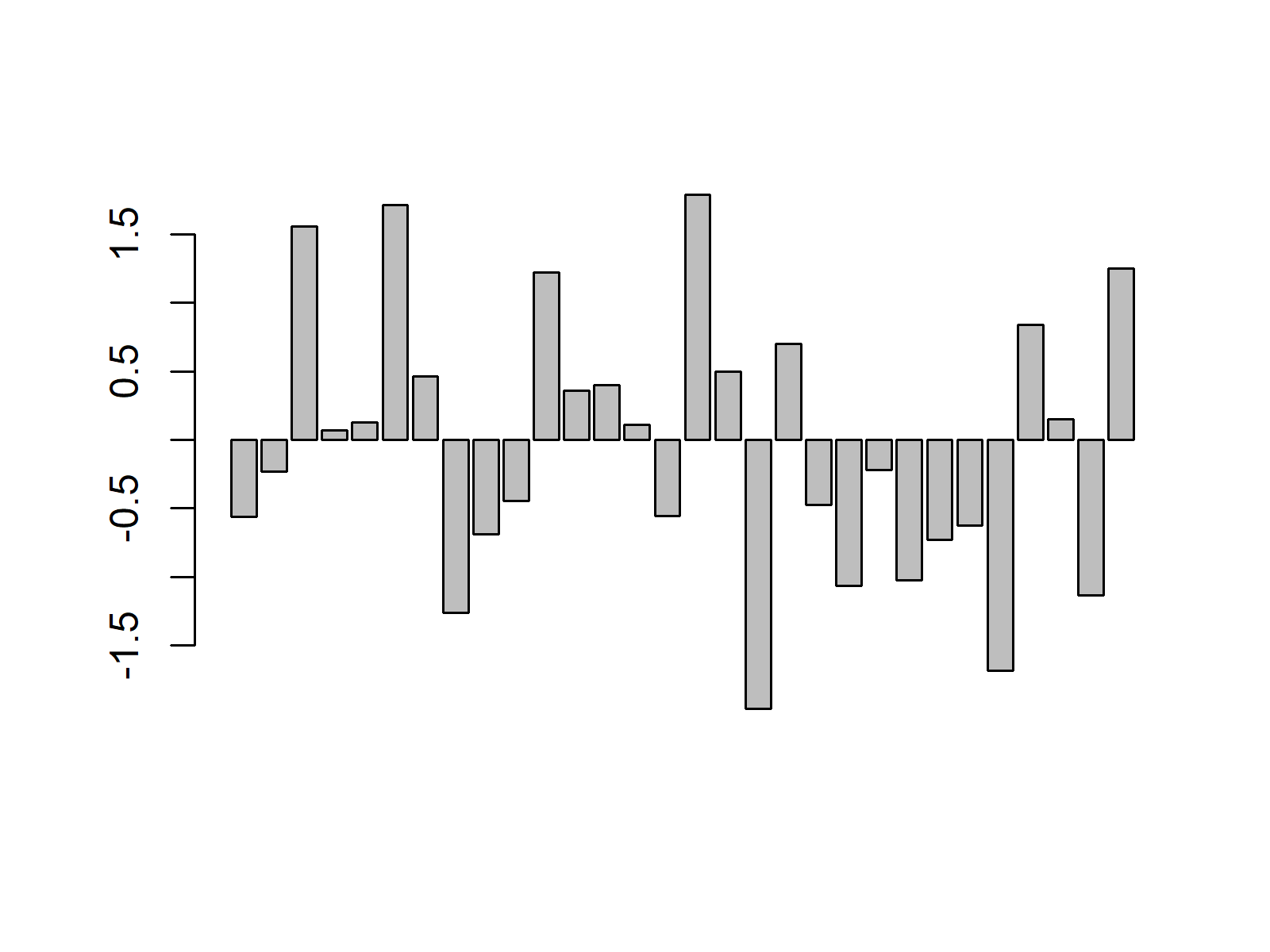Advanced Barplots: Find some advanced barplots below. Click on the images to get more information and example R codes for each of the barplots.

Barplot Resources: Find some further resources on the creation of barplots below.

Barplot Video Tutorial: The following video shows a tutorial on creating barplots in R.

Please accept YouTube cookies to play this video. By accepting you will be accessing content from YouTube, a service provided by an external third party.If you accept this notice, your choice will be saved and the page will refresh.

## Boxplot

Boxplot Definition: A boxplot (or box-and-whisker plot) displays the distribution of a numerical variable based on five summary statistics: minimum non-outlier; first quartile; median; third quartile; and maximum non-outlier. Furthermore, boxplots show the positioning of outliers and whether the data is skewed.

The following R syntax shows how to draw a basic boxplot in R:

`boxplot(x)                           # Draw boxplot in R`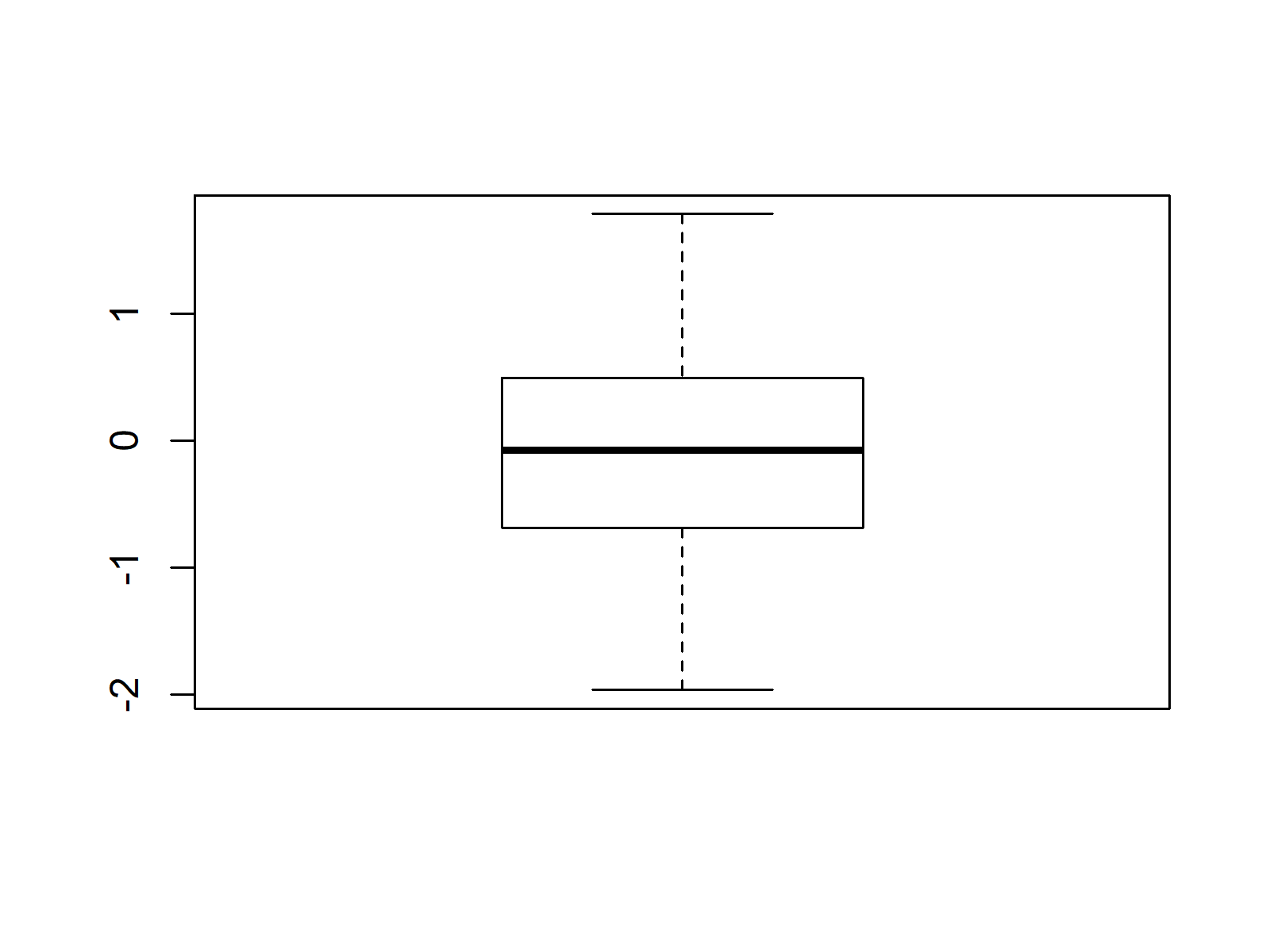Advanced Boxplots: Find some advanced boxplots below. Click on the images to get more information and example R codes for each of the boxplots.

Boxplot Resources: Find some further resources on the creation of boxplots below.

Boxplot Video Tutorial: The following video shows a tutorial on creating boxplots in R.

Please accept YouTube cookies to play this video. By accepting you will be accessing content from YouTube, a service provided by an external third party.If you accept this notice, your choice will be saved and the page will refresh.

## Density Plot

Density Plot Definition: A density plot (or kernel density plot; density trace graph) shows the distribution of a numerical variable over a continuous interval. Peaks of a density plot visualize where the values of numerical variables are concentrated.

The following R syntax shows how to draw a basic density plot in R:

`plot(density(x))                     # Draw density plot in R`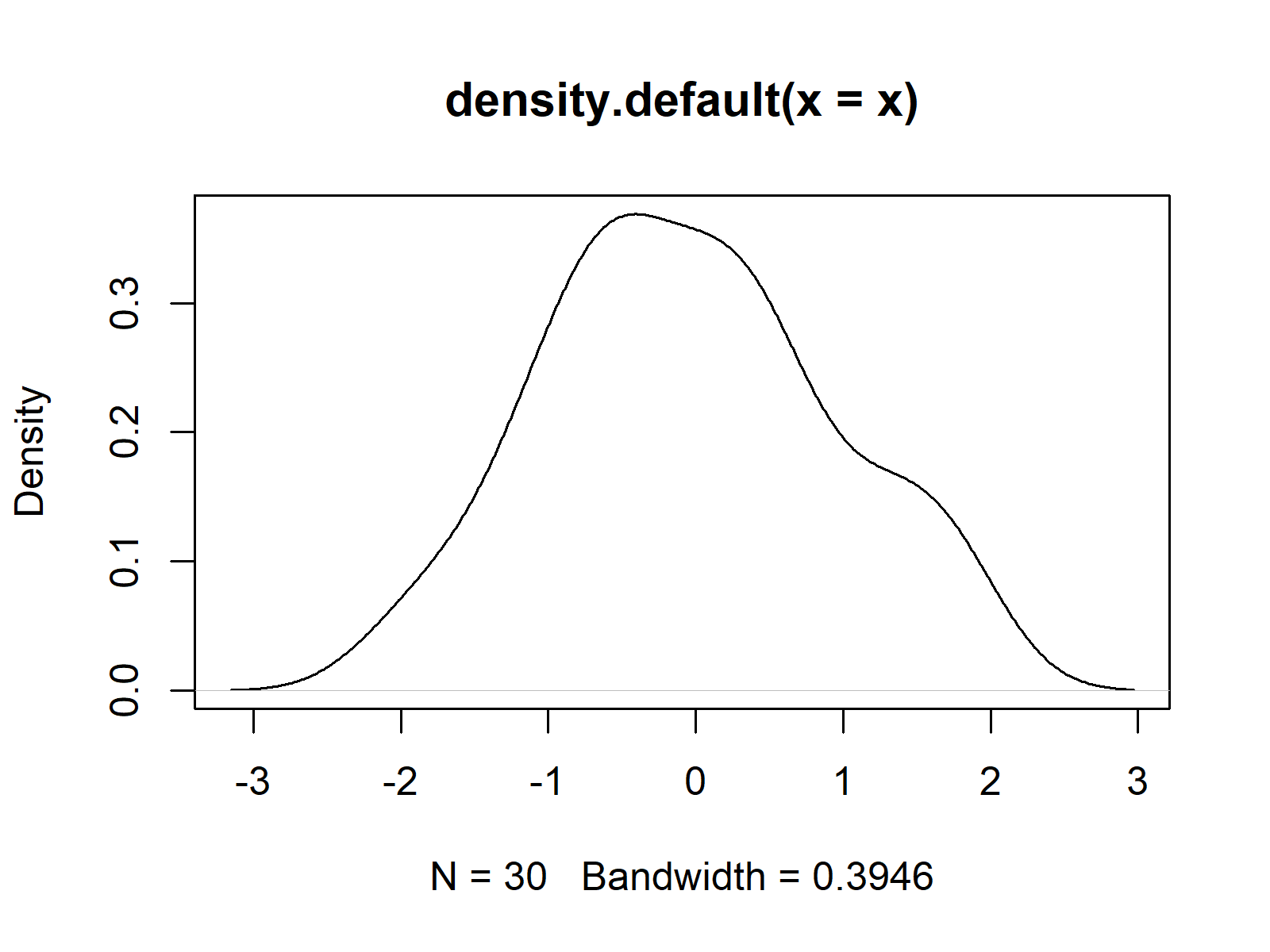Advanced Density Plots: Find some advanced density plots below. Click on the images to get more information and example R codes for each of the density plots.

Density Plot Resources: Find some further resources on the creation of density plots below.

## Heatmap

Heatmap Definition: A heatmap (or shading matrix) visualizes individual values of a matrix with colors. More common values are typically indicated by brighter reddish colors and less common values are typically indicated by darker colors.

The following R syntax shows how to draw a basic heatmap in R:

`heatmap(cbind(x, y))                 # Draw heatmap in R`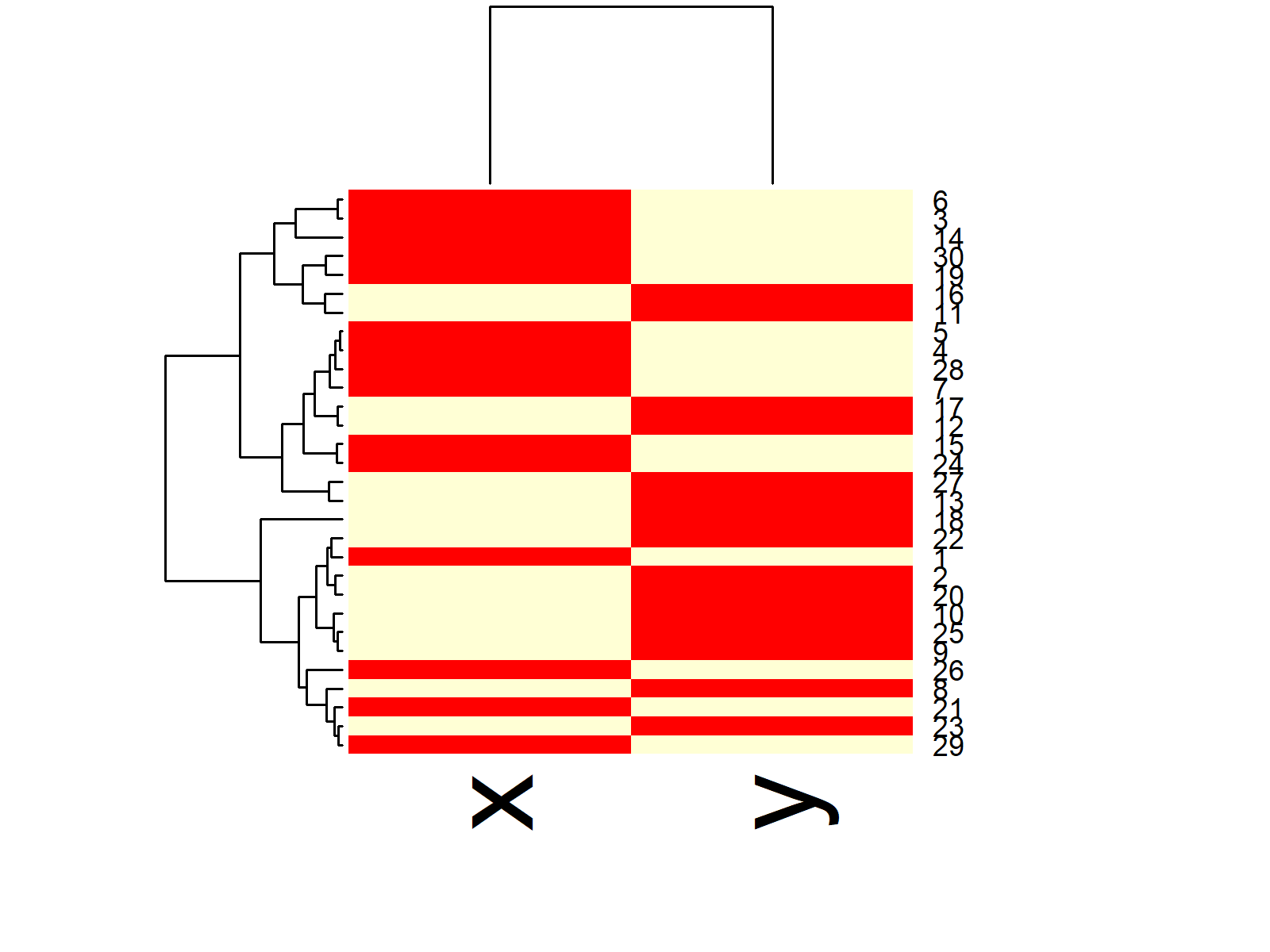Advanced Heatmaps: Find some advanced heatmaps below. Click on the images to get more information and example R codes for each of the heatmaps .

Heatmap Resources: Find some further resources on the creation of heatmaps below.

Heatmap Video Tutorial: The following video shows a tutorial on creating heatmaps in R.

Please accept YouTube cookies to play this video. By accepting you will be accessing content from YouTube, a service provided by an external third party.If you accept this notice, your choice will be saved and the page will refresh.

## Line Plot

Line Plot Definition: A line plot (or line graph; line chart) visualizes values along a sequence (e.g. over time). Line plots consist of an x-axis and a y-axis. The x-axis usually displays the sequence and the y-axis the values corresponding to each point of the sequence.

The following R syntax shows how to draw a basic line plot in R:

`plot(1:length(y), y, type = "l")     # Draw line plot in R`Advanced Line Plots: Find some advanced line plots below. Click on the images to get more information and example R codes for each of the line plots.

Line Plot Resources: Find some further resources on the creation of line plots below.

## Histogram

Histogram Definition: A histogram groups continuous data into ranges and plots this data as bars. The height of each bar shows the amount of observations within each range.

The following R syntax shows how to draw a basic histogram in R:

`hist(x)                              # Draw histogram in R`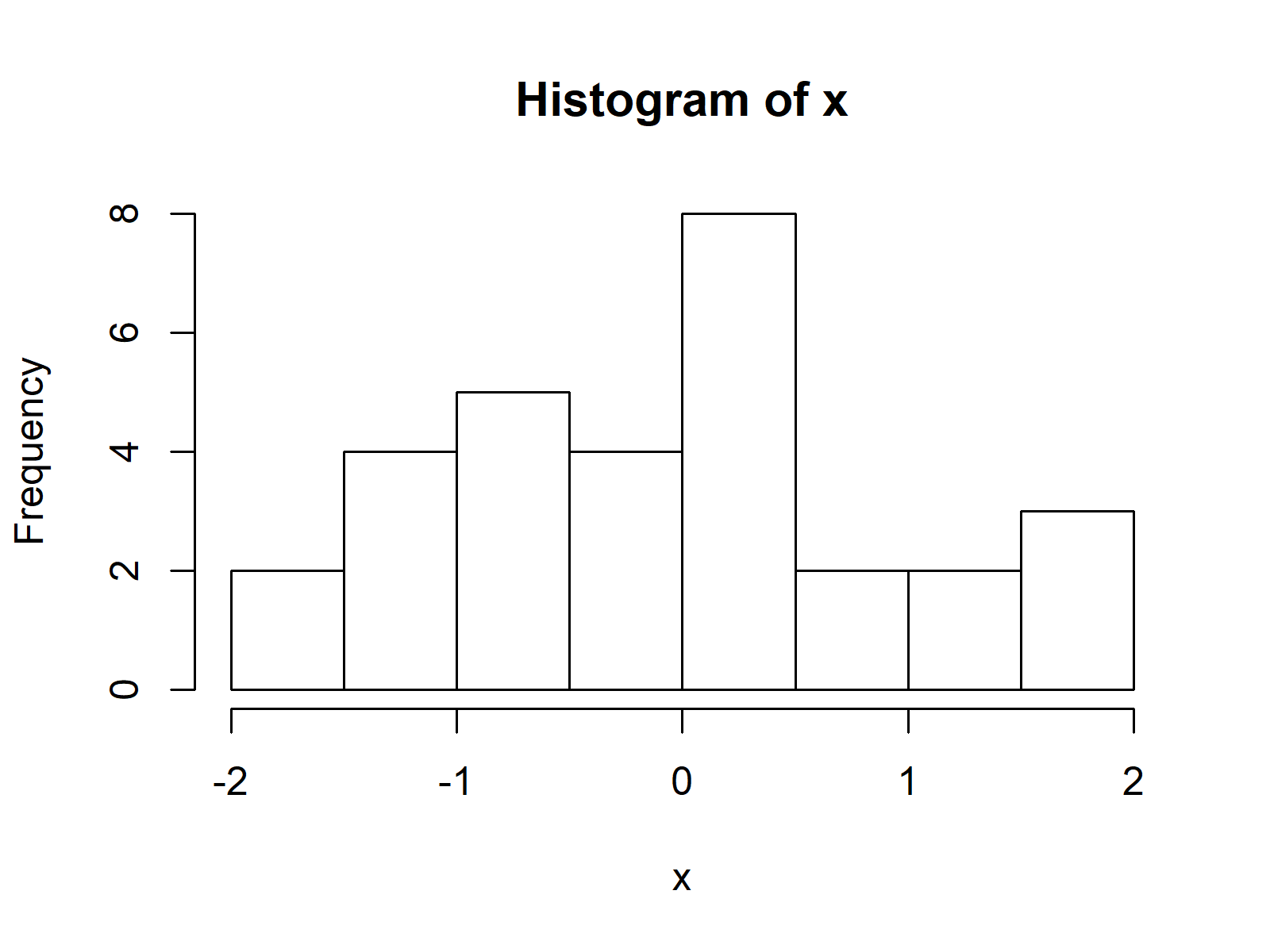Advanced Histograms: Find some advanced histograms below. Click on the images to get more information and example R codes for each of the histograms.

Histogram Resources: Find some further resources on the creation of histograms below.

Histogram Video Tutorial: The following video shows a tutorial on creating histograms in R.

Please accept YouTube cookies to play this video. By accepting you will be accessing content from YouTube, a service provided by an external third party.If you accept this notice, your choice will be saved and the page will refresh.

## Pairs Plot

Pairs Plot Definition: A pairs plot is a plot matrix, consisting of scatterplots for each variable-combination of a data frame.

The following R syntax shows how to draw a basic pairs plot in R:

`pairs(data.frame(x, y))              # Draw pairs plot in R`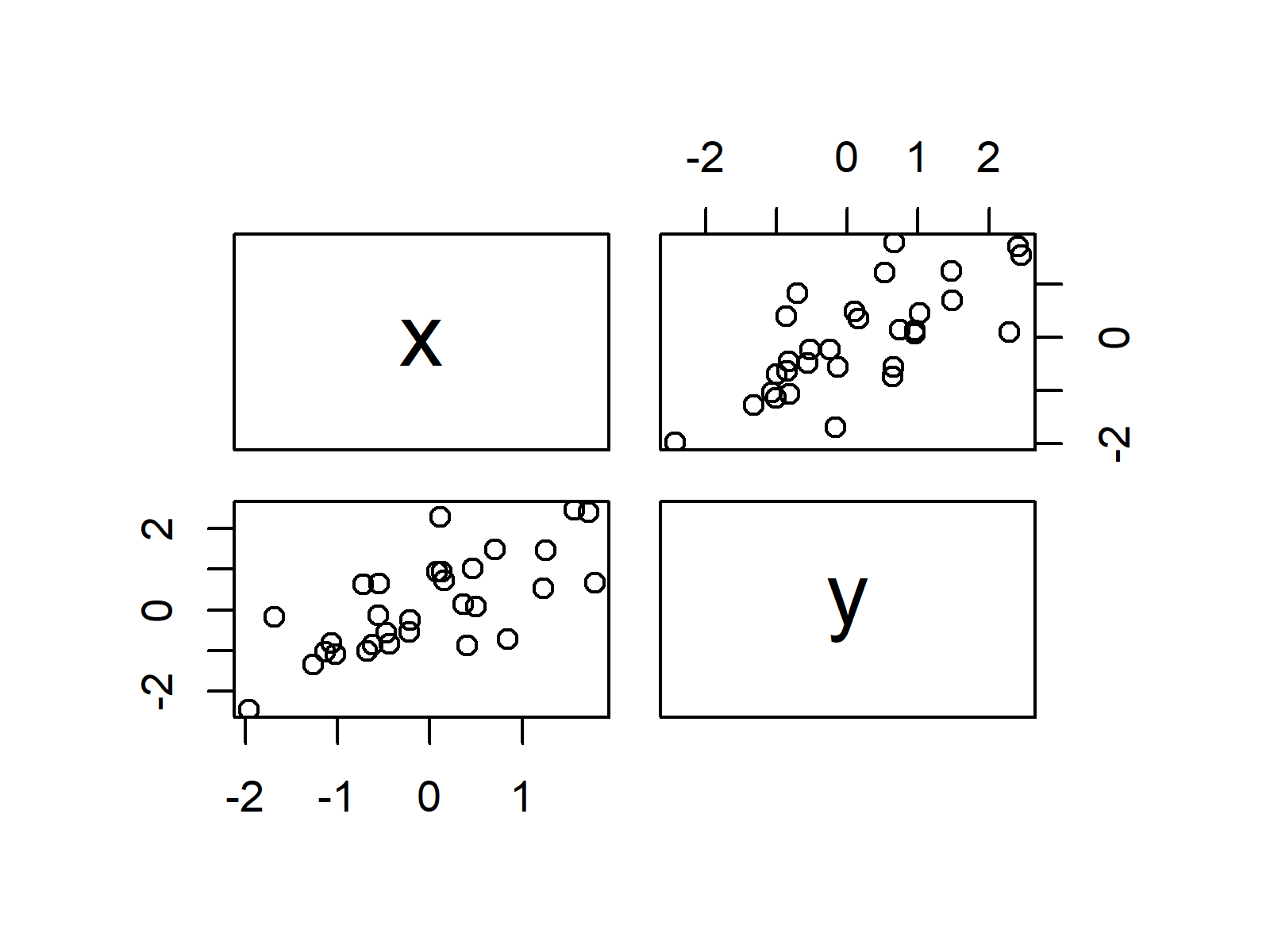Advanced Pairs Plots: Find some advanced pairs plots below. Click on the images to get more information and example R codes for each of the pairs plots.

Pairs Plot Resources: Find some further resources on the creation of pairs plots below.

## Polygon Plot

Polygon Plot Definition: A polygon plot displays a plane geometric figure (i.e. a polygon) within the plot.

The following R syntax shows how to draw a basic polygon plot in R:

```plot(1, 1,                           # Draw polygon plot in R
col = "white",
xlab = "X", ylab = "Y")
polygon(x = c(0.7, 1.3, 1.3, 0.8),
y = c(0.6, 1.0, 1.4, 1.3),
col = "#353436")```Advanced Polygon Plots: Find some advanced polygon plots below. Click on the images to get more information and example R codes for each of the polygon plots.

Polygon Plot Resources: Find some further resources on the creation of polygon plots below.

## QQplot

QQplot Definition: A QQplot (or Quantile-Quantile plot; Quantile-Quantile diagram) determines whether two data sources come from a common distribution. QQplots draw the quantiles of the two numerical data sources against each other. If both data sources come from the same distribution, the points fall on a 45 degree angle.

The following R syntax shows how to draw a basic QQplot in R:

`qqplot(x, y)                         # Draw QQplot in R`Advanced QQplots: Find some advanced QQplots below. Click on the images to get more information and example R codes for each of the QQplots.

QQplot Resources: Find some further resources on the creation of QQplots below.

## Scatterplot

Scatterplot Definition: A scatterplot (or scatter plot; scatter graph; scatter chart; scattergram; scatter diagram) displays two numerical variables with points, whereby each point represents the value of one variable on the x-axis and the value of the other variable on the y-axis.

The following R syntax shows how to draw a basic scatterplot in R:

`plot(x, y)                           # Draw scatterplot in R`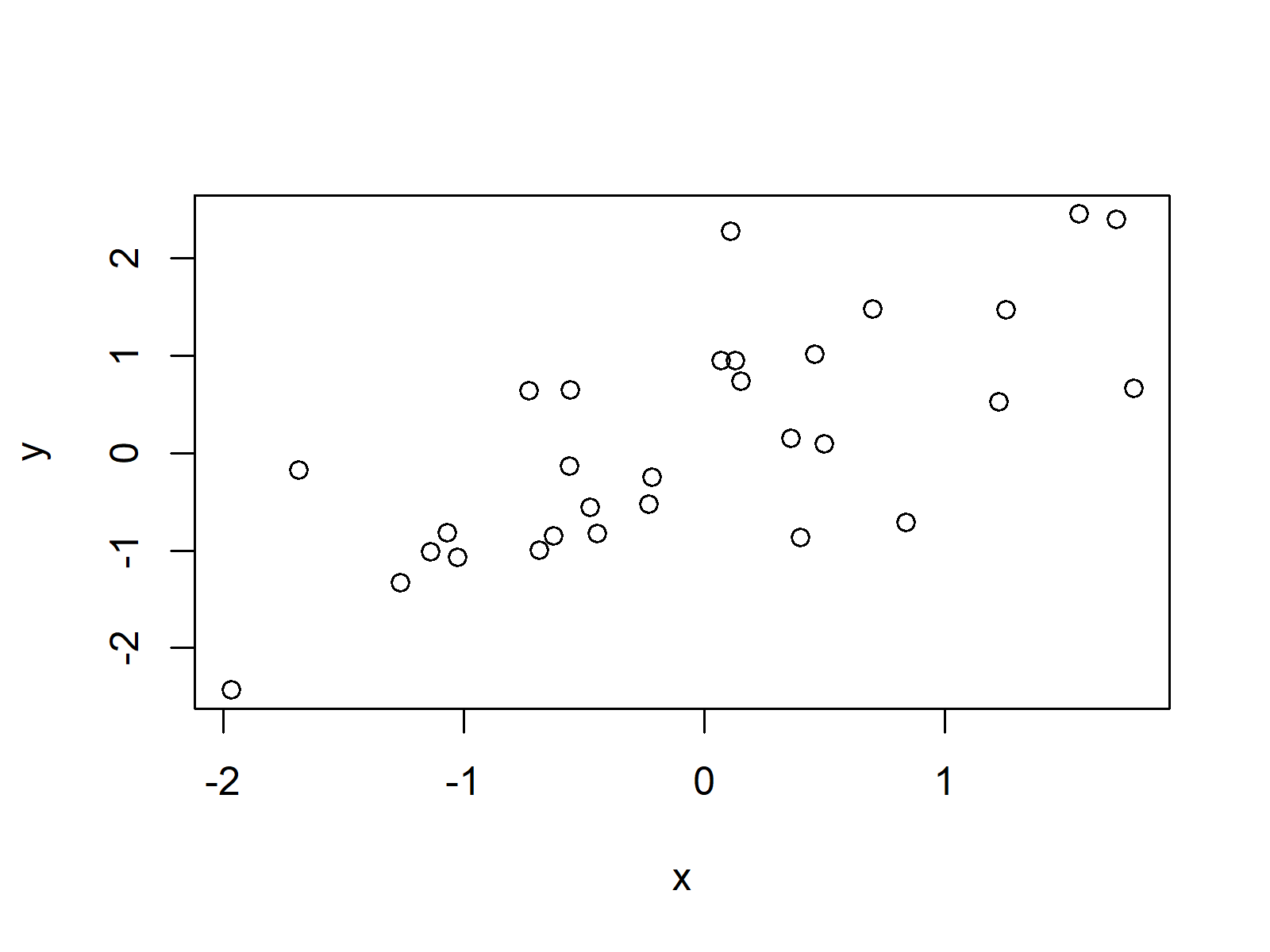Advanced Scatterplots: Find some advanced scatterplots below. Click on the images to get more information and example R codes for each of the scatterplots.

Scatterplot Resources: Find some further resources on the creation of scatterplots below.

## Venn Diagram

Venn Diagram Definition: A venn diagram (or primary diagram; set diagram; logic diagram) illustrates all possible logical relations between certain data characteristics. Each characteristic is represented as a circle, whereby overlapping parts of the circles illustrate elements that have both characteristics at the same time.

The following R syntax shows how to draw a basic venn diagram in R:

```install.packages("VennDiagram")      # Install VennDiagram package
plot.new()                           # Draw empty plot
draw.single.venn(area = 10)          # Draw venn diagram```Advanced Venn Diagrams: Find some advanced venn diagrams below. Click on the images to get further information and example R codes for each of the venn diagrams.

Venn Diagram Resources: Find some further resources on the creation of venn diagrams below.

Venn Diagram Video Tutorial: The following video shows a tutorial on creating venn diagrams in R.

Please accept YouTube cookies to play this video. By accepting you will be accessing content from YouTube, a service provided by an external third party.If you accept this notice, your choice will be saved and the page will refresh.

## General Modification of Plots

In the previous part of this article, I have shown you many different types of plots. However, there are plenty of programming tricks for the modification of plots in general. In the following, you will find a list of tutorials that explain such general modifications of plots in R.

### ggplot2

I have created a detailed introduction to the ggplot2 package, which explains the basic concepts of this library in more detail. You can find the introduction here.

Furthermore, you might have a look at the following list of ggplot2 tutorials, in case you are eager to learn more about certain components of the package.

This tutorial showed an overview of many different graphics and plots of the R programming language. If you are keen to learn more details about the creation of plots in R, I can recommend the following YouTube video of the DataCamp YouTube channel:

Please accept YouTube cookies to play this video. By accepting you will be accessing content from YouTube, a service provided by an external third party.If you accept this notice, your choice will be saved and the page will refresh.

If you would like to learn more about the R programming language in general, you could have a look at the following two links. They show a list of useful R functions…

… and give an overview of all R programming tutorials on this website:

I hope you liked this gallery of R graphics! If you have further questions or any kind of feedback, don’t hesitate to let me know in the comments below.

Subscribe to the Statistics Globe Newsletter

•Jan Schäfer
August 31, 2020 7:12 am

Thanks for the comprehensive introduction into plots!

•August 31, 2020 7:14 am

Hi Jan,

Thanks for the kind words, glad to hear that you liked the introduction!

Regards,

Joachim

•Abdoulaye Sarr
May 22, 2021 1:21 pm

any example on point data on a geographic (country or region ) map. Example temperature or precipitation

•May 25, 2021 6:45 am

Hey Abdoulaye,

I do not have such examples yet, but I’ll put it on my to-do list.

Regards

Joachim

•Dr.D.K.Samuel
June 6, 2021 11:57 am

Extremely useful. Thanks. Will you consider making a tutorial for gganimate please

•June 7, 2021 6:03 am

Hey,

Thanks a lot for the very nice feedback! Yes, this is definitely planned for the future. I’ll keep you updated.

Regards

Joachim

•June 26, 2021 5:15 am

Thank a lot Mr Jaochim, very useful and easy to understand ,it will be very helpful if you add tutorial on time series models ,like GARCh,ARIMA ….
Thanks and regards

•June 26, 2021 6:55 am

Thanks a lot for the kind words!

I have definitely planned to publish more tutorials on time series data in the future. I hope I’ll find the time for it soon 🙂

Regards

Joachim

•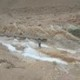Rotem
June 29, 2021 9:37 am

Looking foreward to it 🙂

•June 29, 2021 11:56 am

I’ll keep you updated! 🙂

•smlknk
January 17, 2022 11:07 am

Lad X være som givet ovenfor. Implement´er likelihood funktionen λ 7→ L(λ; 3) i R. Plot funktionen i intervallet [0, 6] og indtegn en lodret streg ved λ = λˆML.

•January 17, 2022 1:16 pm

Hey,

Regards,
Joachim

•Saima
February 21, 2023 12:33 pm

I would like to plot both a histogram for observed data and a fitted Weibull function on the same graph. Can u plz help me?

•Saima
March 6, 2023 10:31 am

I used the following code.
data <- data.frame(x = c(4, 5, 6, 8, 35, 1,23, 4, 3, 27, 3,1, 1, 2, 3,4 ))

ggplot(data, aes(x)) + # Draw histogram with density
geom_histogram(aes(y = ..density..)) +
stat_function(fun = dweibull,
args = list(mean = mean(data\$x),
sd = sd(data\$x)),
col = "#1b98e0",
size = 5)
But I only get the histogram. I don't get the PDF with it.

•March 7, 2023 10:18 am

Hello Saima,

Apparently, the arguments that you should use are shape and scale when it comes to Weibull. They are something to do with the slope and stretch of the curve, see here. Reminding that you should change the inputs of the arguments, you can adapt the following code:

```ggplot(data, aes(x)) + # Draw histogram with density
geom_histogram(aes(y = ..density..)) +
stat_function(fun = dweibull,
args = list(shape = mean(data\$x),
scale = sd(data\$x)),
col = "#1b98e0",
size = 5)```

For further info on plotting continuous distributions via ggplot2, see this.

Regards,
Cansu

•Saima
March 7, 2023 10:29 am

I don’t know how to thank you. You are such a wonderful guy. Many thanks.

•March 7, 2023 11:00 am